# 【Unity】如何优雅地移动物体-8个方法

2022-09-23 08:36:25给我丶鼓励

Unity 引擎也提供了众多的方法，每个开发者的使用习惯也各不相同，所以往往不是很清楚在这种场景下哪种方式最好的或者最有效的。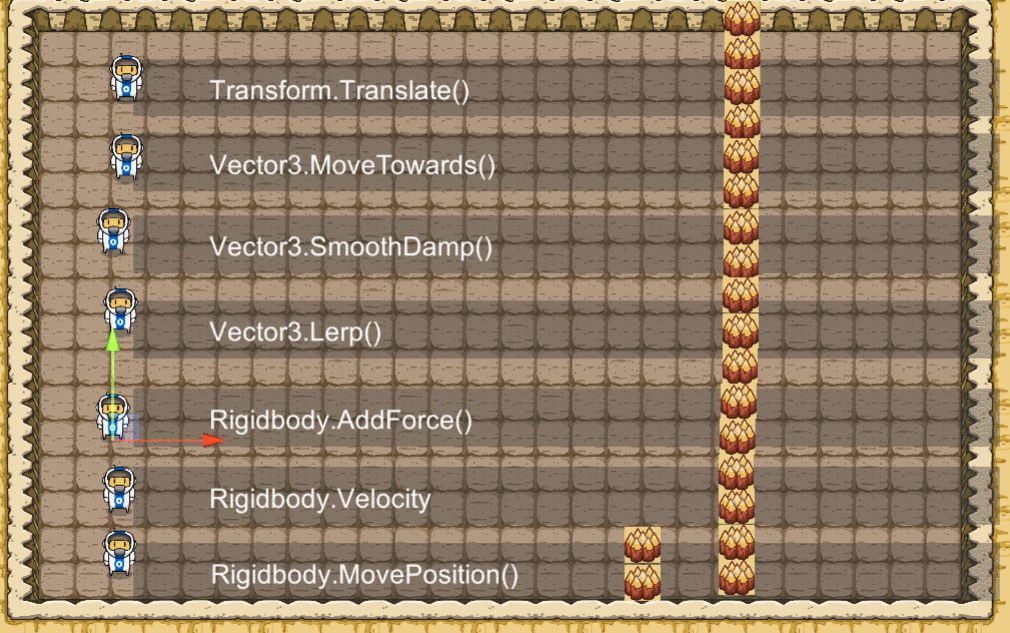## 向某个方向移动

### Transform.Position

``````transform.position = new Vector3(2, 1, 0);
``````

``````    void Update()
{

var dir = new Vector3(0.02f, 0, 0);
transform.position += dir;
}
``````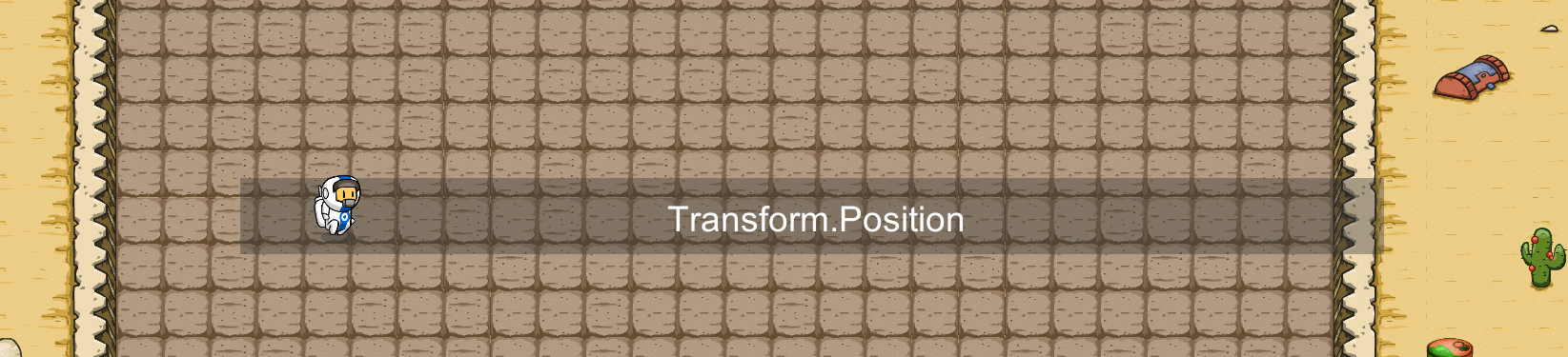### Transform.Translate()

``````      void Update()
{

var dir = new Vector3(0.02f, 0, 0);
transform.Translate(dir);
}
``````

`````` public void Translate(Vector3 translation, [DefaultValue("Space.Self")] Space relativeTo)
{

if (relativeTo == Space.World)
this.position += translation;
else
this.position += this.TransformDirection(translation);
}
``````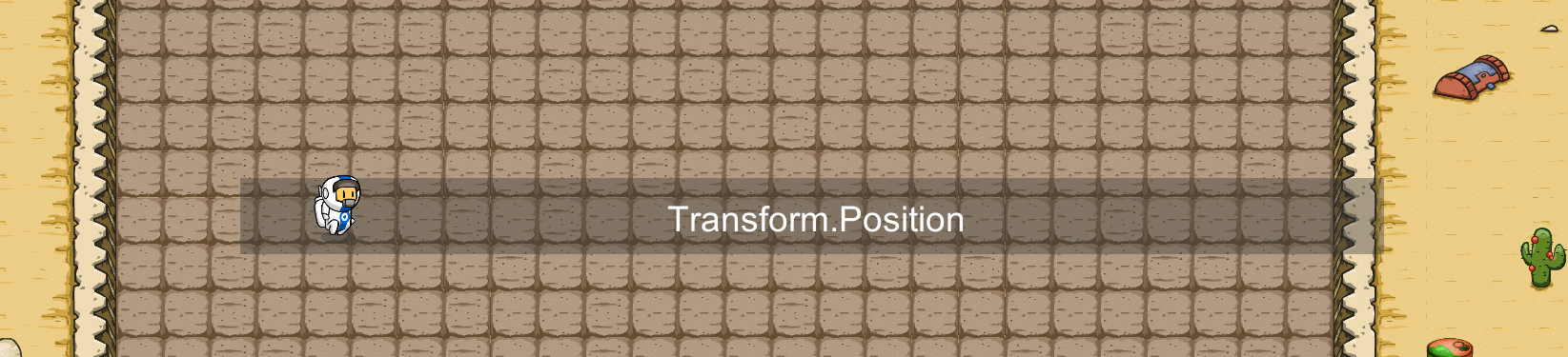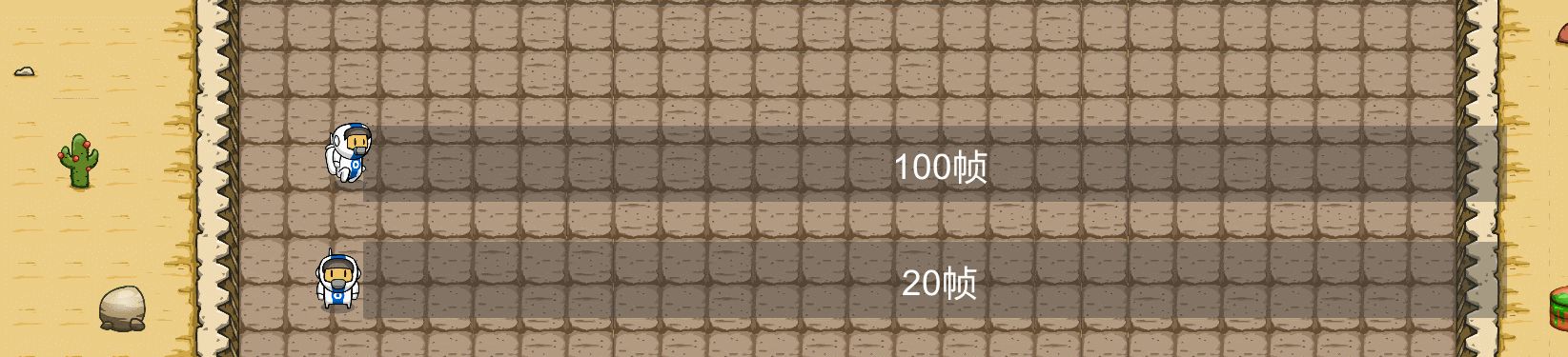``````void Update()
{

var dir = new Vector3(2f, 0, 0)*time;
transform.Translate(dir);
}
``````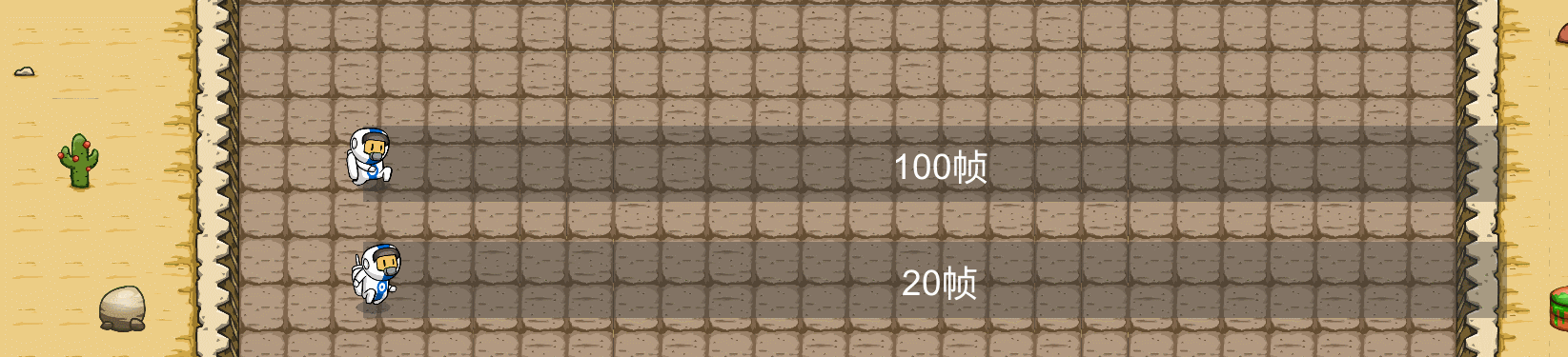``````public float speed = 2;
void Update()
{

var dir = new Vector3(2,0,0)
transform.Translate(dir.normalized * speed * Time.deltaTime);
}
``````

## 移动到指定位置

1. 速度：物体通过特定速度向目标移动。
2. 时间：物体在时间内到达目标。

### Vector3.MoveTowards()：固定速度

``````public Vector3 targetPosition;
public float speed=10;
void Update()
{

transform.position = Vector3.MoveTowards(transform.position, targetPosition, speed * Time.deltaTime);
}
``````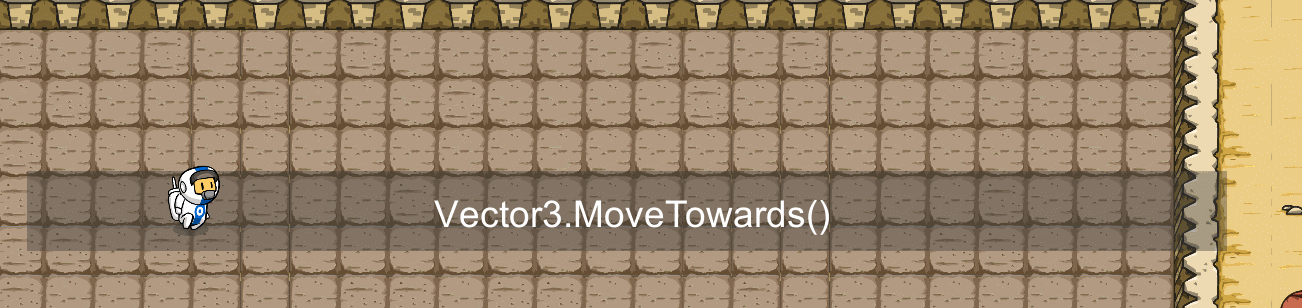### Vector3.SmoothDamp()：平滑移动

``````public Vector3 targetPosition;
public float smoothTime = 0.5f;
public float speed = 10;
Vector3 velocity ;
void Update()
{

transform.position = Vector3.SmoothDamp(transform.position, targetPosition, ref velocity, smoothTime, speed);
}
``````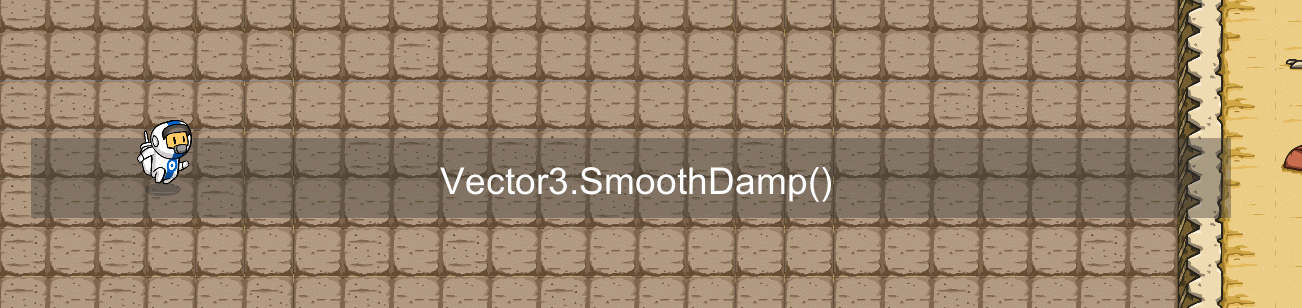### Vector3.Lerp()：线性时间移动

`````` // 终点
public Vector3 targetPosition;
// 开始位置
public Vector3 startPosition;
// 持续时间
public float lerpDuration = 4;
// 记录运行时间
private float _timeElapsed = 0;

void Start()
{

startPosition = transform.position;
}

void Update()
{

// 记录下一个位置
Vector3 valueToLerp;
_timeElapsed += Time.deltaTime;
if (_timeElapsed < lerpDuration)
{

valueToLerp  = Vector3.Lerp(startPosition, targetPosition, _timeElapsed / lerpDuration);
}
else
{

valueToLerp = targetPosition;
}
transform.position = valueToLerp;
}
``````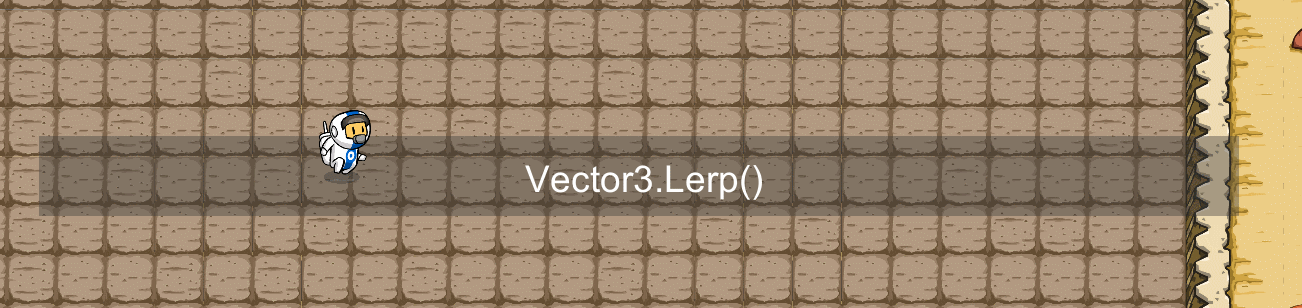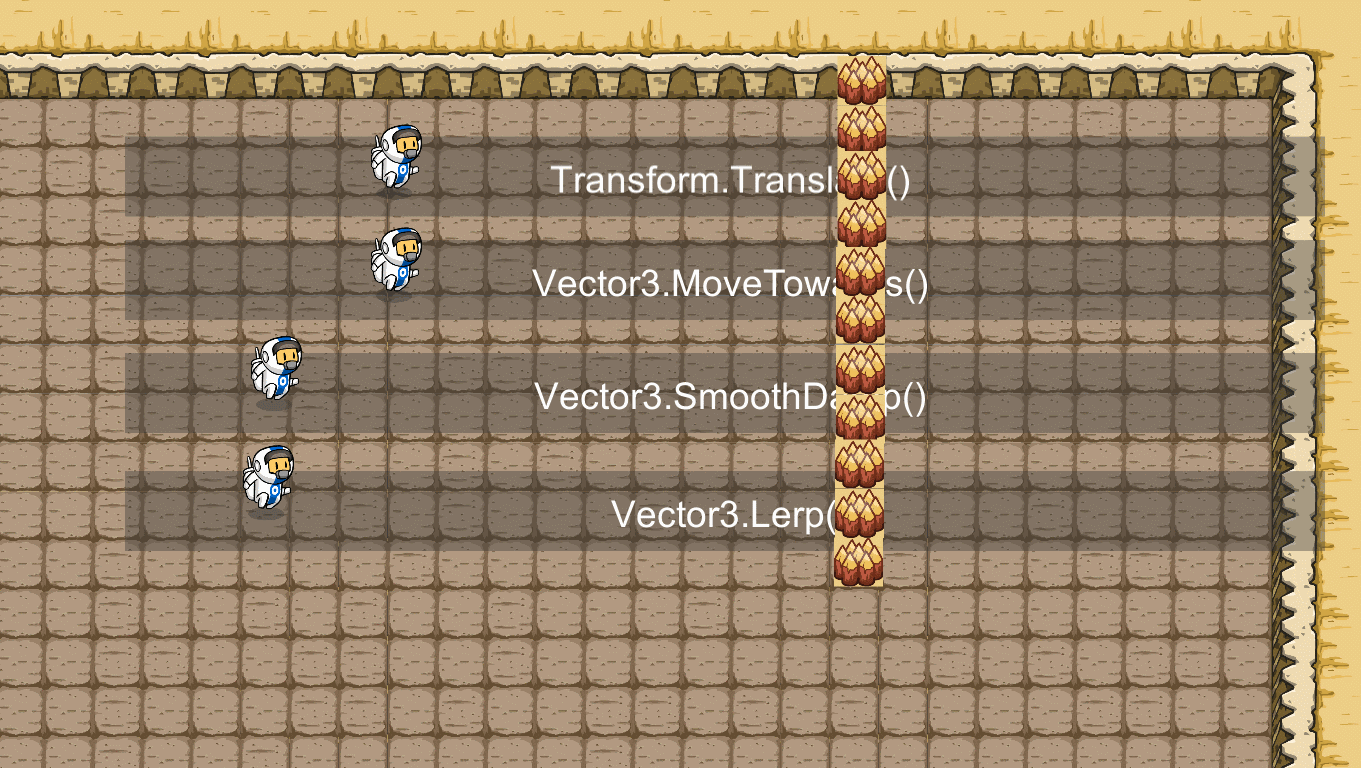## 物理引擎移动

``````    // 赋予200的力
public float force = 200;
private Rigidbody2D _rigidbody2D;
// 移动方向
private Vector3 dir = Vector3.right;

void Start()
{

_rigidbody2D = GetComponent<Rigidbody2D>();
}

``````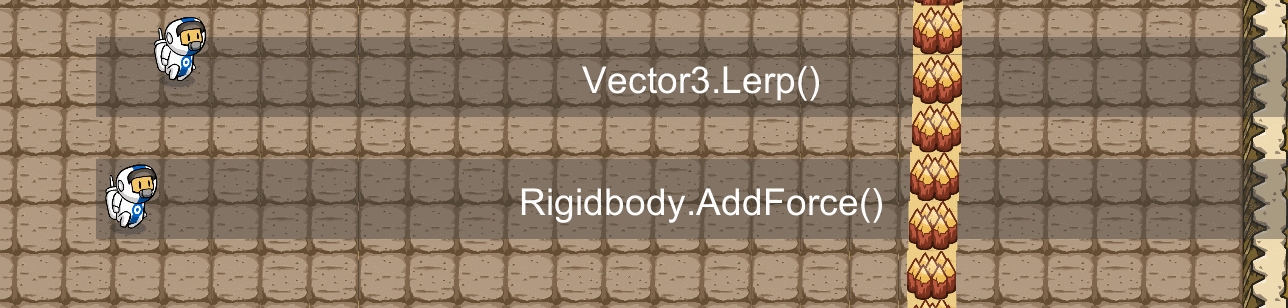`````` // 赋予2的力
public float force = 2;
private Rigidbody2D _rigidbody2D;
// 移动方向
private Vector3 dir = Vector3.right;
void Start()
{

_rigidbody2D = GetComponent<Rigidbody2D>();
}

void FixedUpdate()
{

}
``````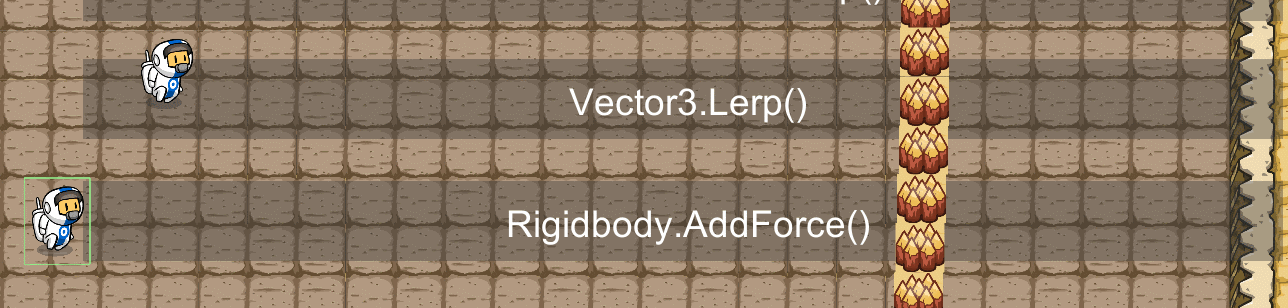### Rigidbody.Velocity

``````    public float speed = 10;
private Rigidbody2D _rigidbody2D;
// 移动方向
private Vector3 dir = Vector3.right;

void Start()
{

_rigidbody2D = GetComponent<Rigidbody2D>();
}

void FixedUpdate()
{

_rigidbody2D.velocity = dir * speed;
}
``````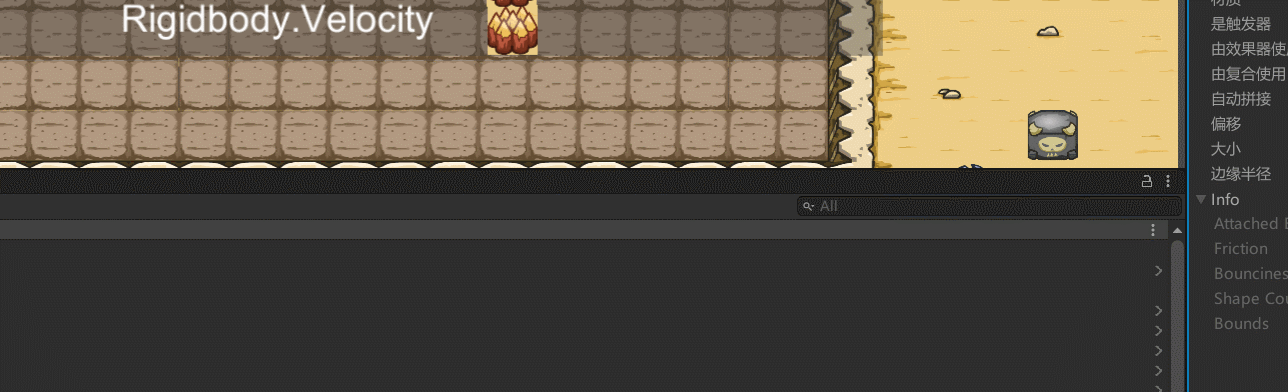### Rigidbody.MovePosition()

``````	public float speed = 10;
private Rigidbody2D _rigidbody2D;
// 移动方向
private Vector3 dir = Vector3.right;

void Start()
{

_rigidbody2D = GetComponent<Rigidbody2D>();
}

private void FixedUpdate()
{

var positon = dir * (speed * Time.deltaTime);
_rigidbody2D.MovePosition(transform.position + positon);
}
``````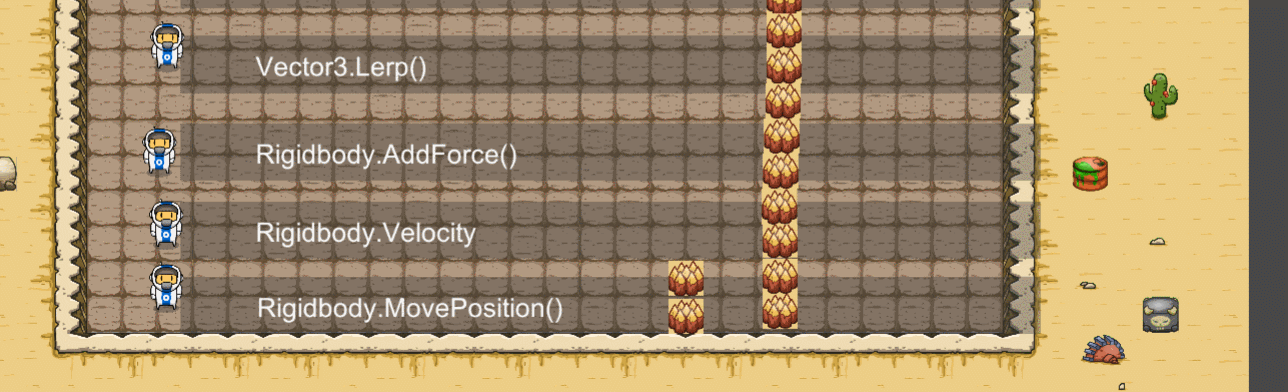https://blog.csdn.net/GG_and_DD/article/details/126917358